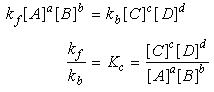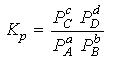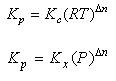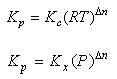1800-1023-196

+91-120-4616500

# Law of Mass Action & Equilibrium Constant

## Law of Mass Action & Equilibrium Constant

On the basis of observations of many equilibrium reactions, two Norwegian chemists Goldberg and Waage suggested (1864) a quantitative relationship between the rates of reactions and the concentration of the reacting substances. This relationship is known as law of mass action. It states that

“The rate of a chemical reaction is directly proportional to the product of the molar concentrations of the reactants at a constant temperature at any given time.”

The molar concentration i.e. number of moles per litre is also called active mass. It is expressed by enclosing the symbols of formulae of the substance in square brackets. For example, molar concentration of A is expressed as [A].

Consider a simple reversible reaction

ab + bB ⇌ cC + dD (At a certain temperature)

According to law of mass action

Rate of forward reaction ∞ [A]a [B]b = kf [A]a [B]b

Rate of backward reaction ∞ [C]c [D]d = kb [C]c [D]d

At equilibrium,

Rate of forward reaction = Rate of backward reactionWhere, is called equilibrium constant.

In terms of partial pressures, equilibrium constant is denoted by Kp andIn terms of mole fraction, equilibrium constant is denoted by Kx andRelation between Kp, Kc and KxDn = number of moles of gaseous products – number of moles of gaseous reactants in chemical equation.

As a general rule, the concentration of pure solids and pure liquids are not included when writing an equilibrium equation.

 Value of Dn Relation between Kp and Kc Units of Kp Units of Kc 0 Kp = Kc No unit No unit >0 Kp > Kc (atm)Dn (mole l–1)Dn

## NEET & AIIMS Exam Sample Papers

 AIIMS SAMPLE PAPERS View More NEET SAMPLE PAPERS View More